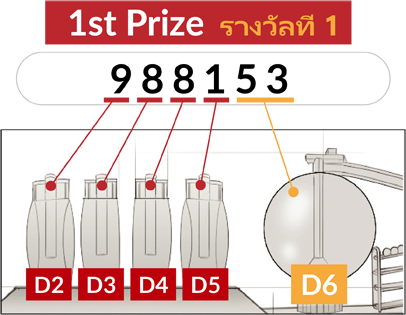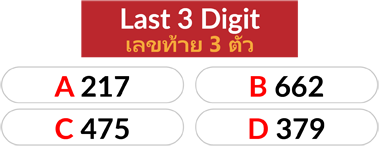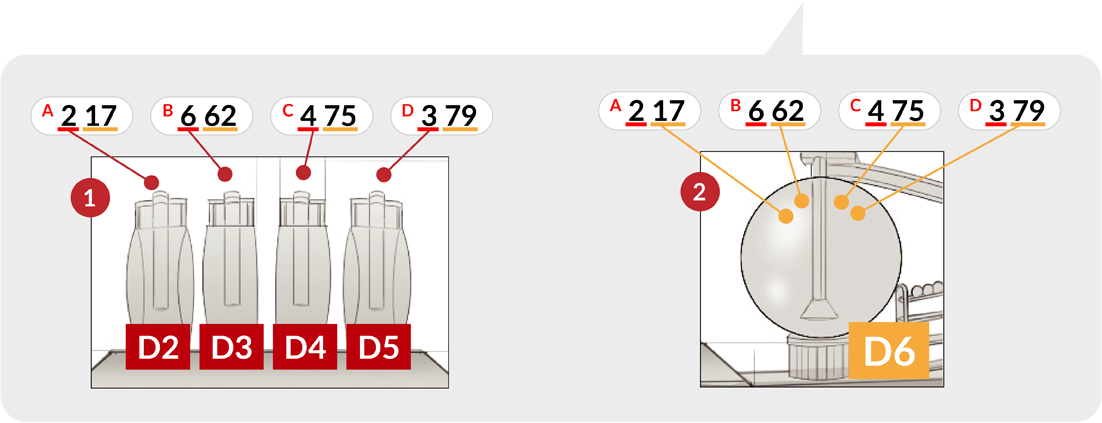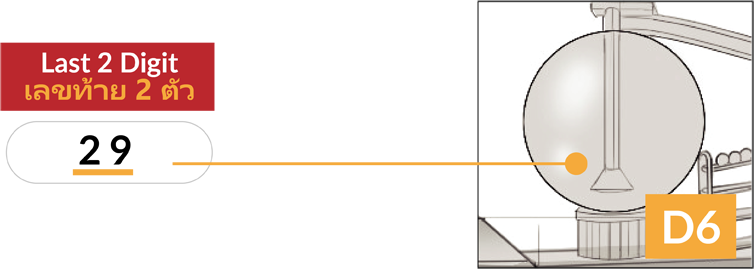﻿

### 6D RESULT NUMBER

Once the D1 has decided on the draw sequence for 6D. Then we will start to draw the 6D result.

1. D2 will draw the first digit of 6D.
2. D3 will draw the second digit of 6D.
3. D4 will draw the third digit of 6D.
4. D5 will draw the fourth digit of 6D.
5. D6 will draw the last two digits of 6D.### 3D RESULT NUMBER

Once the D1 has decided on the draw sequence for 3D.
Then we will start to draw the 3D result. Total we have 4 set of 3D results.

1. D2 will draw the first digit of the first 3D set.
2. D3 will draw the first digit of the second 3D set.
3. D4 will draw the first digit of the third 3D set.
4. D5 will draw the first digit of the fourth 3D set.
5. D6 will draw the last two digits for first, second, third and fourth 3D set.### 2D RESULT NUMBER

Once the D1 has decided on the draw sequence for 2D. Then we will start to draw the 2D result.
For 2D result, we only use D6 to draw the two digits for 2D result.